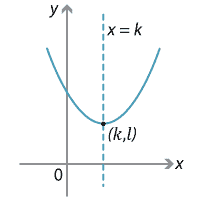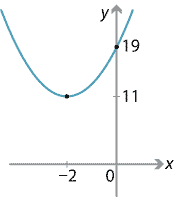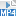### The axis of symmetry

All parabolas have exactly one axis of symmetry (unlike a circle, which has infinitely many axes of symmetry). If the vertex of a parabola is $$(k,l)$$, then its axis of symmetry has equation $$x=k$$.Detailed description of diagram

We can find a simple formula for the value of $$k$$ in terms of the coefficients of the quadratic. As usual, we complete the square:

\begin{align*} y &= ax^2 + bx + c\\ &= a\Big[x^2 + \dfrac{b}{a}x + \dfrac{c}{a}\Big]\\ &= a\Big[\Big(x+\dfrac{b}{2a}\Big)^2 + \dfrac{c}{a} - \Big(\dfrac{b}{2a}\Big)^2\Big]. \end{align*}

We can now see that the $$x$$-coordinate of the vertex is $$-\dfrac{b}{2a}$$. Thus the equation of the axis of symmetry is

$x = -\dfrac{b}{2a}.$

We could also find a formula for the $$y$$-coordinate of the vertex, but it is easier simply to substitute the $$x$$-coordinate of the vertex into the original equation $$y=ax^2+bx+c$$.

#### Example

Sketch the parabola $$y=2x^2+8x+19$$ by finding the vertex and the $$y$$-intercept. Also state the equation of the axis of symmetry. Does the parabola have any $$x$$-intercepts?

#### Solution

Here $$a=2$$, $$b=8$$ and $$c=19$$. So the axis of symmetry has equation $$x=-\dfrac{b}{2a}=-\dfrac{8}{4}=-2$$. We substitute $$x=-2$$ into the equation to find $$y = 2\times (-2)^2 + 8\times (-2) + 19 = 11$$, and so the vertex is at $$(-2,11)$$. Finally, putting $$x=0$$ we see that the $$y$$-intercept is 19.

There are no $$x$$-intercepts.Detailed description of diagram

##### Exercise 3

A parabola has vertex at $$(1,3)$$ and passes through the point $$(3,11)$$. Find its equation.

Screencast of exercise 3Next page - Content - The quadratic formula and the discriminant